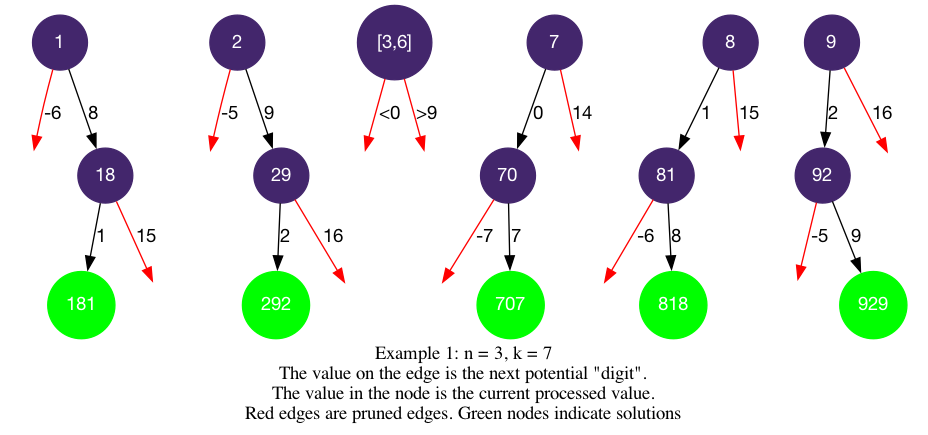# LeetCode Numbers With Same Consecutive Differences Solution

Given two integers n and k, return an array of all the integers of length `n` where the difference between every two consecutive digits is `k`. You may return the answer in any order.

Note that the integers should not have leading zeros. Integers as `02` and `043` are not allowed.

Example 1:

Input: `n = 3, k = 7`

Output: `[181,292,707,818,929]`

Explanation: Note that `070` is not a valid number, because it has leading zeroes.

Example 2:

Input: `n = 2, k = 1`

Output: `[10,12,21,23,32,34,43,45,54,56,65,67,76,78,87,89,98]`

Constraints:

• `2 <= n <= 9`
• `0 <= k <= 9`

## Solution

We want to build up the numbers from the leftmost digit to the rightmost. Since `n` is >= 2 and there should not be leading zeros, we will start from one digit (1-9) and call our backtracking helper to construct the next digits. Here, our `path` (`num` in the implementation) will store an integer instead of a list to save space and time.

We want to apply backtracking1 template. We fill in the logic:

• `is_leaf`: `start_index == n`, when the constucted number contains `n` digits.
• `get_edges`: the next digit can be `cur_digit +- k`
• `is_valid`: `cur_digit + k <= 9` and `cur_digit - k >= 0` to avoid the next digit being out of bound. Note that in the implementation, if `k=0` then `cur_digit+k == cur_digit - k`, we must avoid the duplicate by checking whether `k=0`.

#### Implementation

``````1def numsSameConsecDiff(self, n: int, k: int) -> List[int]:
2    def dfs(start_index, num):
3        if start_index == n:  # num is n digits, add to ans
4            ans.append(num)
5            return
6        cur_digit = num % 10        # current digit
7        if (cur_digit - k >= 0):
8            dfs(start_index + 1, num * 10 + (cur_digit - k))
9        if (cur_digit + k <= 9 and k != 0): # avoid duplicate when k = 0
10            dfs(start_index + 1, num * 10 + (cur_digit + k))
11    ans = []
12    for i in range(1, 10):  # first digit can be 1-9
13        dfs(1, i)
14    return ans``````